# 3D Coordinate Conversion VI

## LabVIEW 2018 Help

Edition Date: March 2018
Part Number: 371361R-01
View Product Info

### DOWNLOAD (Windows Only)LabVIEW 2016 HelpLabVIEW 2017 HelpLabVIEW 2018 HelpLabVIEW 2019 HelpLabVIEW 2020 Help

Owning Palette: Geometry VIs

Requires: Full Development System

Converts coordinates between the Cartesian, spherical, and cylindrical coordinate systems. Wire data to the Axis 1 input to determine the polymorphic instance to use or manually select the instance.

Use the pull-down menu to select an instance of this VI.

 Select an instance 3D Coordinate Conversion (Array)3D Coordinate Conversion (Scalar)

## 3D Coordinate Conversion (Array)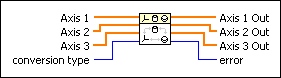Axis 1 specifies the X-coordinates in a Cartesian coordinate system, rho-coordinates in a cylindrical coordinate system, or radius-coordinates in a spherical coordinate system.Axis 2 specifies the Y-coordinates in a Cartesian coordinate system, theta-coordinates in a cylindrical coordinate system, or theta-coordinates in a spherical coordinate system.Axis 3 specifies the Z-coordinates in a Cartesian coordinate system, z-coordinates in a cylindrical coordinate system, or phi-coordinates in a spherical coordinate system.conversion type specifies the type of conversion to perform.

 0 Cartesian to spherical (default) 1 spherical to Cartesian 2 Cartesian to cylindrical 3 cylindrical to Cartesian 4 spherical to cylindrical 5 cylindrical to spherical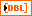Axis 1 Out returns the coordinates on the first axis in the new coordinate system.Axis 2 Out returns the coordinates on the second axis in the new coordinate system.Axis 3 Out returns the coordinates on the third axis in the new coordinate system.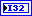error returns any error or warning from the VI. You can wire error to the Error Cluster From Error Code VI to convert the error code or warning into an error cluster.

## 3D Coordinate Conversion (Scalar)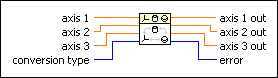axis 1 specifies the X-coordinate in a Cartesian coordinate system, rho-coordinate in a cylindrical coordinate system, or radius-coordinate in a spherical coordinate system.axis 2 specifies the Y-coordinate in a Cartesian coordinate system, theta-coordinate in a cylindrical coordinate system, or theta-coordinate in a spherical coordinate system.axis 3 specifies the Z-coordinate in a Cartesian coordinate system, z-coordinate in a cylindrical coordinate system, or phi-coordinate in a spherical coordinate system.conversion type specifies the type of conversion to perform.

 0 Cartesian to spherical (default) 1 spherical to Cartesian 2 Cartesian to cylindrical 3 cylindrical to Cartesian 4 spherical to cylindrical 5 cylindrical to spherical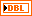axis 1 out returns the coordinate on the first axis in the new coordinate system.axis 2 out returns the coordinate on the second axis in the new coordinate system.axis 3 out returns the coordinate on the third axis in the new coordinate system.error returns any error or warning from the VI. You can wire error to the Error Cluster From Error Code VI to convert the error code or warning into an error cluster.

## 3D Coordinate Conversion Details

The following illustrations show a point P in different three-dimensional coordinate systems: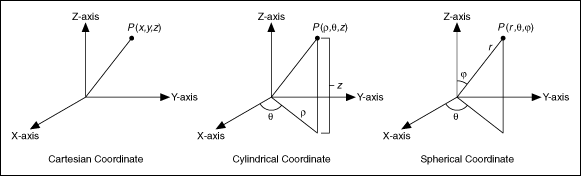The Cartesian, or rectangular, coordinate system is the most widely used coordinate system. The cylindrical coordinate system is a generalization of two-dimensional polar coordinates to three dimensions. The following equations describe the relationship between a Cartesian coordinate and a cylindrical coordinate:

x =· cos, y =· sin, z = zis the radial coordinate, and(–<) is the azimuthal coordinate.

The spherical coordinate system is a system of curvilinear coordinates that is natural for describing positions on a sphere. The following equations describe the relationship between a Cartesian coordinate and a spherical coordinate:

x = r · sin· cos, y = r · sin· sin, z = r · cosr is the distance from point P to the origin.(–<) is the azimuthal angle, and(0) is the polar angle.

Not Helpful